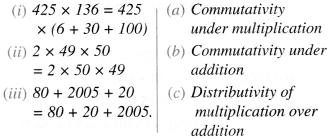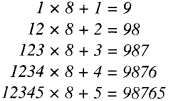# NCERT Solutions for Class 6 Maths Chapter 2 Whole Numbers Ex 2.1, Ex 2.2, Ex. 2.3

NCERT Class Six Mathematics Chapter 2 which is the whole number that is being provided to the students through exercise solutions. There are mainly three exercises in this chapter Exercise 2.1, 2.2 and 2.3 Solutions of all these exercises have been provided to you, you should solve the questions of Mathematics first and then you can take the help of our solution.

 Board CBSE and State Board Exam Textbook NCERT Class Class 6 Subject Maths Chapter Chapter 2 Chapter Name Whole Numbers Exercise Ex 2.1,  Ex 2.2,  Ex 2.3 Number of Questions Solved 20 Category NCERT Mathematics Solutions Class 6

Contents

## NCERT Solutions for Class 6 Maths Chapter 2 Whole Numbers Ex 2.1

Question 1.

### Write the next three natural numbers after 10999.

Solution :
The next three natural numbers after 10999 are 11000, 11001 and 11002.

Question 2.

### Write the three whole numbers occurring just before 10001.

Solution :
The three whole numbers occurring just before 10001 are 10000, 9999 and 9998.

Question 3.

### Which is the smallest whole number?

Solution :
0 is the smallest whole number.

Question 4.

### How many whole numbers are there between 32 and 53?

Solution :
There are 20 whole numbers between 32 and 53.
These are 33, 34, 35, 36, 37, 38, 39, 40, 41, 42,43, 44, 45, 46, 47,48, 49, 50, 51 and 52.

Question 5.

### Write successor of: (a) 2440701 (b) 100199 (c) 1099999 (d) 2345670

Solution :
(a) The successor of 2440701 is 24,40,702.
(b) The successor of 100199 is 1,00,200.
(c) The successor of 1099999 is 11,00,000.
(d) The successor of 2345670 is 23,45,671.

Question 6.

### Write the predecessor of: (a) 94 (b) 10000 (c) 208090 (d) 7654321.

Solution :
(a) The predecessor of 94 is 93.
(b) The predecessor of 10000 is 9,999.
(c) The predecessor of 208090 is 2,08,089.
(d) The predecessor of 7654321 is 76,54,320.

Question 7.

### In each of the following pairs of numbers, the state which the whole number is on the left of the other number on the number line. Also write them with the appropriate sign (>, <) between them. (a) 530, 503 (b) 370, 307 (c) 98765, 56789 (d) 9830415, 10023001.

Solution :
(a) The whole number 503 is on the left of the whole number 530 on the number line. So, 503 < 530.
(b) The whole number 307 is on the left of the whole number 370 on the number line. So, 307 < 370.
(c) The whole number 56789 is on the left of the whole number 98765 on the number line. So, 56789 < 98765. ;
(d) The whole number 9830415 is on the left of the whole number 10023001 on the number line. So, 98,30,415 < 100,23,001.

Question 8.

### Which of the following statements are true (T) and which are false (F)? (a) Zero is the smallest natural number. (b) 400 is the predecessor of 399. (c) Zero is the smallest whole number. (d) 600 is the successor of 599. (e) All natural numbers are whole numbers. (f) All whole numbers are natural numbers, (g) The predecessor of a two digit number is never a single digit number. (h) 1 is the smallest whole number. (i) The natural number 1 has no predecessor, (j) The whole number 1 has no predecessor. (k) The whole number 13 lies between 11 and 12. (l) The whole number 0 has no predecessor. (m) The successor of a two digit number is always a two digit number.

Solution :
(a) This statement is false (F).
(b) This statement is false (F).
(c) This statement is true (T).
(d) This statement is true (T).
(e) This statement is true (T).
(f) This statement is false (F).
(g) This statement is false (F).
(h) This statement is false (F).
(i) This statement is true (T).
(j) This statement is false (F).
(k) This statement is false (F).
(l) This statement is true (T).
(m) This statement is false (F).

## NCERT Solutions for Class 6 Maths Chapter 2 Whole Numbers Ex 2.2

Question 1.

### Find the sum by suitable rearrangement : (a) 837 + 208 + 363 (b) 1962 + 453 + 1538 + 647.

Solution :
(a) 837 + 208 + 363
= 837 + 363 + 208 = (837 + 363) + 208 = 1200 + 208 = 1408
(b) 1962+ 453+ 1538 + 647
= 1962 + 1538 + 453 + 647 = (1962 + 1538) + (453 + 647)
= 3500+ 1100 = 4600.

Question 2.

### Find the product by a suitable rearrangement : (a) 2 × 1768 × 50 (b) 4 × 166 × 25 (c) 8 × 291 × 125 (d) 625 × 279 × 16 (e) 285 × 5 × 60 (f) 125 × 40 × 8 × 25.

Solution :
(a) 2 × 1768 × 50
= 2 × 50 × 1768 = (2 × 50) × 1768 = 100 × 1768= 1,76,800
(b) 4 × 166 × 25 = 4 × 25 × 166 = (4 × 25) × 166 = 100 × 166 = 16,600
(c) 8 × 291 × 125 = 8 × 125 × 291
= (8 × 125) × 291 = 1000 × 291 =2,91,000
(d) 625 × 279 × 16 = 625 × 16 × 279
= (625 × 16) × 279 = 10000 ×279 = 27,90,000
(e) 285 × 5 × 60 = 285 × (5 × 60) = 285 × 300
= 85,500
(f) 125 × 40 × 8 × 25 = (125 × 40) × (8 × 25) = 5000 × 200 = 10,00,000.

Question 3.

### Find the value of the following : (a) 297 × 17+297 × 3 (b) 54279 × 92 + 8 × 54279 (c) 81265 × 169 – 81265 × 69 (d) 3845 × 5 × 782 + 769 × 25 × 218.

Solution :
(a) 297 × 17 + 297 × 3 = 297 × (17+ 3)
= 297 × 20 = 5940
(b) 54279 × 92 + 8 × 54279
= 54279 × 92 + 54279 × 8 = 54279 × (92 + 8)
= 54279 × 100 = 54,27,900
(c) 81265 × 169-81265 × 69
= 81265 × (169-69)
= 81265 × 100 = 81,26,500
(d) 3845 × 5 × 782 + 769 × 25 × 218
= 3845 × 5 × 782 + 769 × 5 × 5 × 218 = 3845 × 5 × 782 + (769 × 5) × 5 × 218 = 3845 × 5 × 782 + 3845 × 5 × 218 = 3845 × 5 × (782 + 218)
= 3845 × 5 × 1000 = 19225 × 1000 = 1,92,25,000.

### Question 4. Find the product, using suitable properties : (a) 738 × 103 (b) 854 × 102 (c) 258 × 1008 (d) 1005 × 168.

Solution :
(a) 738 × 103
= 738 × (100+ 3)
= 738 × 100 + 738 × 3 = 73,800 + 2,214 = 76,014
(b) 854 × 102
= 854 × (100 + 2)
= 854 × 100 + 854 × 2 = 85,400+ 1,708 = 87,108
(c) 258 × 1008
= 258 × (1000+ 8)
= 258 × 1000 + 258 × 8 = 2,58,000 + 2,064 = 2,60,064
(d) 1005 × 168
= 168 × 1005
= 168 × (1000+ 5)
= 168 × 1000+ 168 × 5
= 1,68,000 + 840 = 1,68,840.

Question 5.

### A taxi driver filled his car petrol tank with 40 liters of petrol on Monday. The next day he filled the tank with 50 liters of petrol. If the petrol costs ₹ 44 per liter, how much did he spend all on petrol?

Solution :
Petrol filled on Monday = 40 litres
Petrol filled the next day = 50 litres
∴ Total petrol filled on the two days = 40 litres + 50 litres = 90 litres
∴ Cost of petrol per litre = ₹ 44
∴ Cost of 90 litres of 7 petrol = ₹ 44 × 90 = ? 3960.

Question 6.

### A vendor’supplies 32 liters of milk to a hotel in the morning and 68 liters of milk in the evening. If the milk costs ₹ 15 per liter, how much money is due to the vendor per day?

Solution :
Milk supplied in the morning = 32 liters
Milk supplied in the evening = 68 liters
∴ Milk supplied per day = 32 litres + 68 litres = 100 litres
Cost of milk per liter = ₹ 15
Money due to the vendor per day = Cost of
100 litres of milk = ₹ 15 × 100 = ₹ 1500.

Question 7.

### Match the following :Solution :## NCERT Solutions for Class 6 Maths Chapter 2 Whole Numbers Ex 2.3

Question 1.

### Which of the following will not represent (a) 1 + 0 (b) 0 × 0 (c) 02 (d) 10102

Solution :
(a) 1 + 0 = 1 ≠ 0
(b) 0 × 0 = 0
(c) 02
(d) 10102=02=0
Hence, (a) 1 + 0 will not represent zero.

Question 2.

### If the product of two whole numbers is zero, can we say that one or both of them will be zero? Justify through examples.

Solution :
Yes! For example :
2 × 0 = 0
0 × 3 = 0
0 × 0 = 0.

Question 3.

### If the product of two whole numbers is 1. can we say that one or both of them will be 1? Justify through examples.

Solution :
Both of them must be ‘ 1’ as 1 × 1 = 1.

Question 4.

### Find using distributive property : (a) 728 × 101 (b) 5437 × 1001 (c) 824 × 25 (d) 4275 × 125 (e) 504 × 35

Solution :Question 5.

### Study the pattern :Write the next two steps. Can you say how the pattern works ? (Hint : 12345 =11111 + 1 1 11 + 111 + 11 + 1). Sol. Next two steps are as follows :

Solution :
Next two steps are as follows :
123456 x 8 + 6 = 987654
1234567 x 8 + 7 = 9876543.
Working of the pattern• ncert solutions for class 6 maths chapter 2
• class 6 maths chapter 2
• ncert maths book class 6 chapter 2 solutions
• class 6 maths chapter 2 exercise 2.2 solutions
• ncert class 6 maths chapter 2
• class 6 maths chapter 2 pdf
• maths class 6 chapter 2
• class 6 maths chapter 2 question answer
• ncert solutions for class 6 maths chapter 2 try these
• class 6 maths chapter 2 exercise 2.1 solutions
• class 6 maths chapter 2 exercise 2.2
• class 6 chapter 2 maths
• chapter 2 maths class 6
• class 6 maths chapter 2 solution
• chapter 2 class 6 maths
• ncert maths class 7 chapter 2 exercise 2.1 question 6
• ncert solutions for class 6 maths chapter 2 pdf
• class 6 maths chapter 2 exercise 2.3
• ncert maths class 6 chapter 2
• ncert solutions for class 6 maths chapter 2 exercise 2.2
• ncert class 6 maths chapter 2 pdf
• maths chapter 2 class 6
• maths class 6 chapter 2 exercise 2.2
• class 6 maths chapter 2 exercise 2.1
• ncert solutions class 6 maths chapter 2 exercise 2.2
• class 6 maths chapter 2 exercise 2.3 solutions
ncert class 6 maths chapter 2 exercise 2.2
ncert solutions for class 6 maths chapter 2 exercise 2.3
ncert maths class 6 chapter 2 exercise 2.2
ncert class 6 maths chapter 2 worksheet
error: